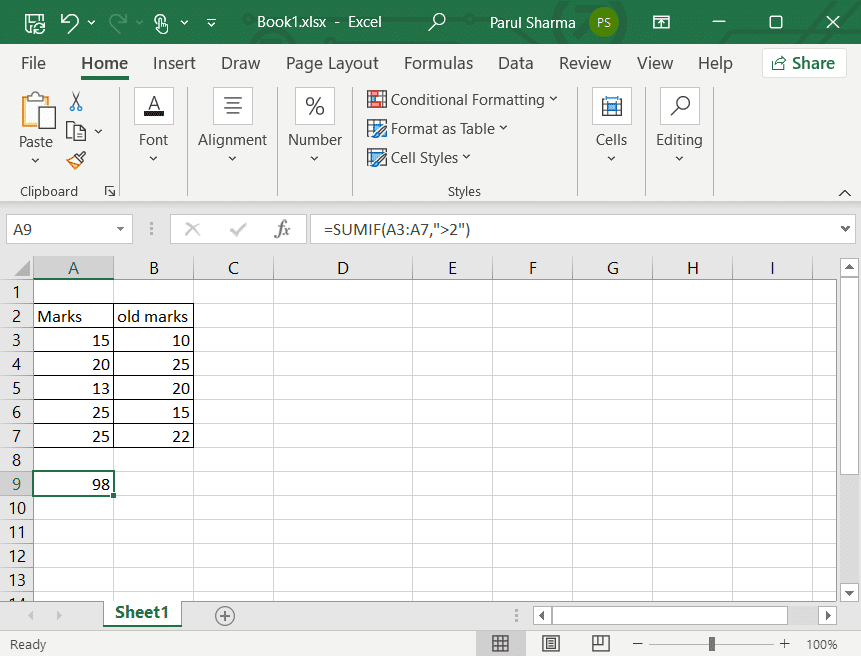# 10 Functions of MS Excel that Everyone Should Know

Microsoft Excel is hands-down one of the best tools for people who work with a huge amount of data every morning. It makes managing everything in one place a cool breeze. Moreover, you can use different functions and formulas to solve complex mathematical problems within a few seconds only. But if you are someone who is just beginning with basic excel formulas then you might be wondering about what 10 functions of MS Excel are and how to use them. So, keep on reading to know more.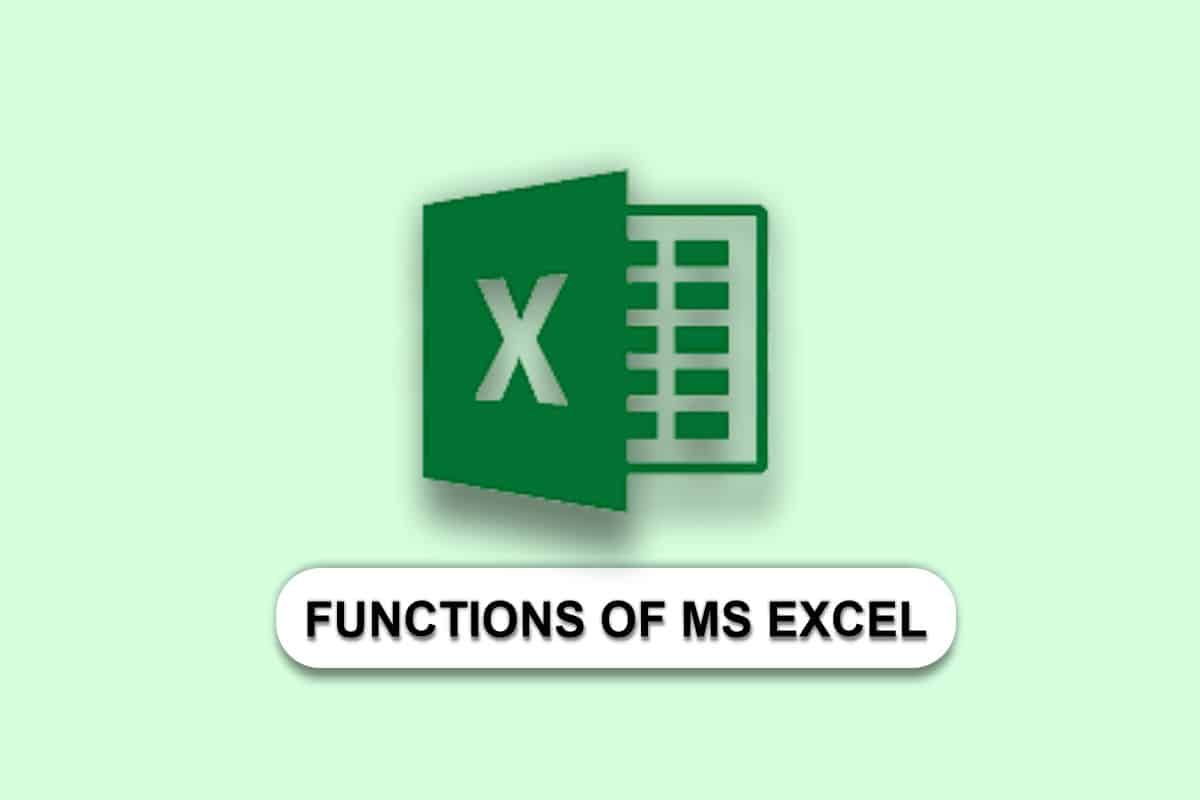## 10 Functions of MS Excel that Everyone Should Know

If MS Excel is your go-to tool, then you are doing great because it cannot get any better than this. Despite its popularity, there are still a lot of people like freshers who are still struggling with Excel functions and formulas. This article will help you with learning 10 functions of MS Excel so you can make things easier than ever.

Some of the important Excel functions include SUMIF, COUNTIF, CEILING, REPLACE, SUBSTITUTE, NOW, TODAY, MODULUS, POWER.

### What are the TOP 10 Excel Formulas?

Let us first take a look at what are the top 10 excel formulas. In simple words, MS Excel formulas are expressions that operate on values within the specified range of cells. Using these formulas, you can easily do so much more than basic subtraction and addition. You can easily use these formulas to carry out big tasks within a few seconds despite the massive amount of data. This sums up what are the top 10 Excel formulas.

Also Read: How to Export Trello Board to Excel

### Top 10 Excel Functions

Depending on your requirement, there are plenty of formulas that you can use to execute an operation. Let us take a look at the top 10 excel functions:

#### 1. CEILING

This function is used to round up a number to its nearest multiple of significance.

=CEILING(number,significance)

• Number– The value you want to round.
• Significance– The multiple to which you want the number to round up to.#### 2. FLOOR

This among the rest 10 functions of MS Excel is the opposite of FLOOR() function. This function rounds a particular number down towards zero to its nearest multiple of significance.

=FLOOR(number,significance)

• Number– It is the numeric value you want to round.
• Significance– It is the multiple to which you want to round the number.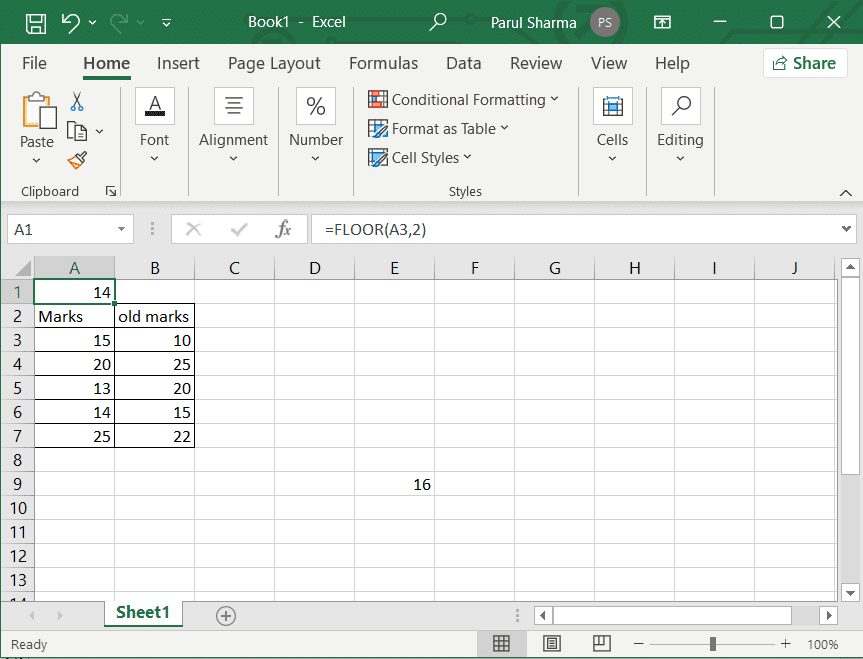#### 3. REPLACE

This function is used to replace a part of any text string with another text string.

=REPLACE(old_text, start_num, num_chars, new_text)

• Old_text– This is the text in which you wish to replace some characters.
• Start_num– This refers to the position of the character present in the old_text which you want to replace.
• Num_chars– This is the number of characters in old_text that you want REPLACE() to replace.
• New_text– This is the text that will replace characters in old_text.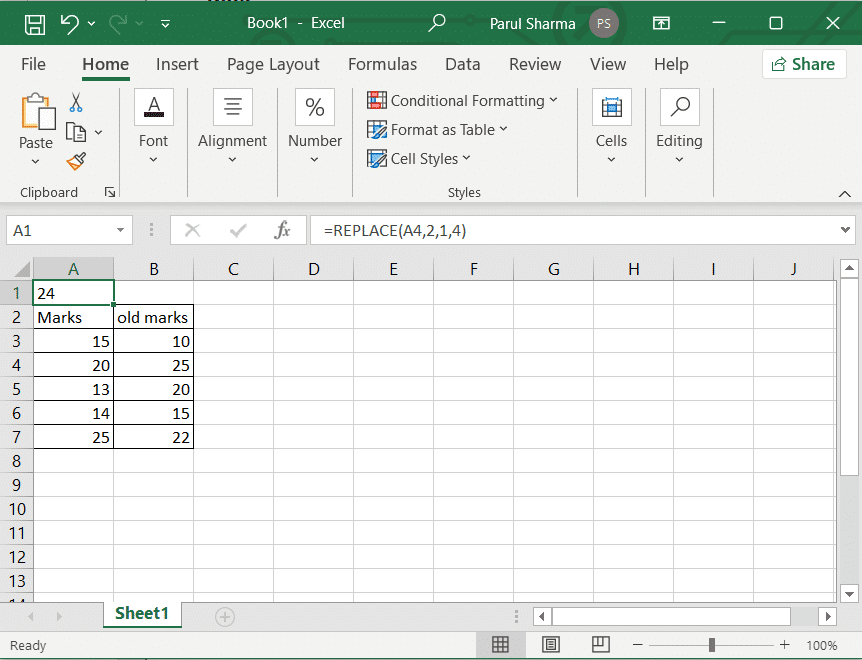Also Read: How to Swap Columns or Rows in Excel

#### 4. SUBSTITUTE

Another one of the basic 10 functions of MS Excel is SUBSTITUTE function. It is used for replacing the existing text with a new text in a text string.

=SUBSTITUTE(text, old_text, new_text, [instance_num])

• Text– It is the text in which you want to substitute characters.
• Old_text– This refers to the text you want to replace.
• New_text– It is the new text that will replace the old text.
• Instance_num– This is optional. It merely specifies which occurrence of old_text you wish to substitute with new_text. For instance, if you use instance_num, only that particular instance of old_text is replaced.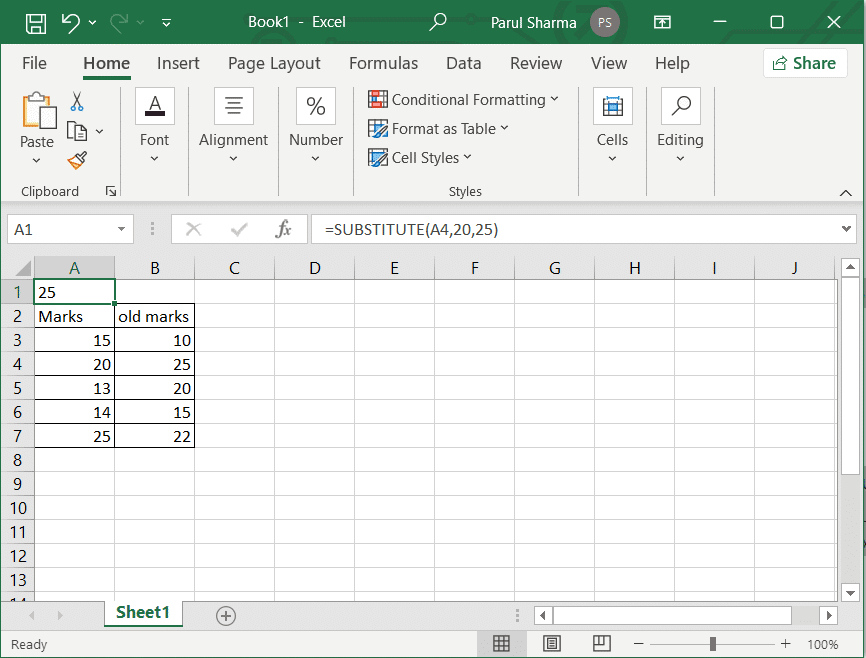#### 5. NOW

This function gives the current system date and time. However, it will change according to the system data and time on your device.

=NOW()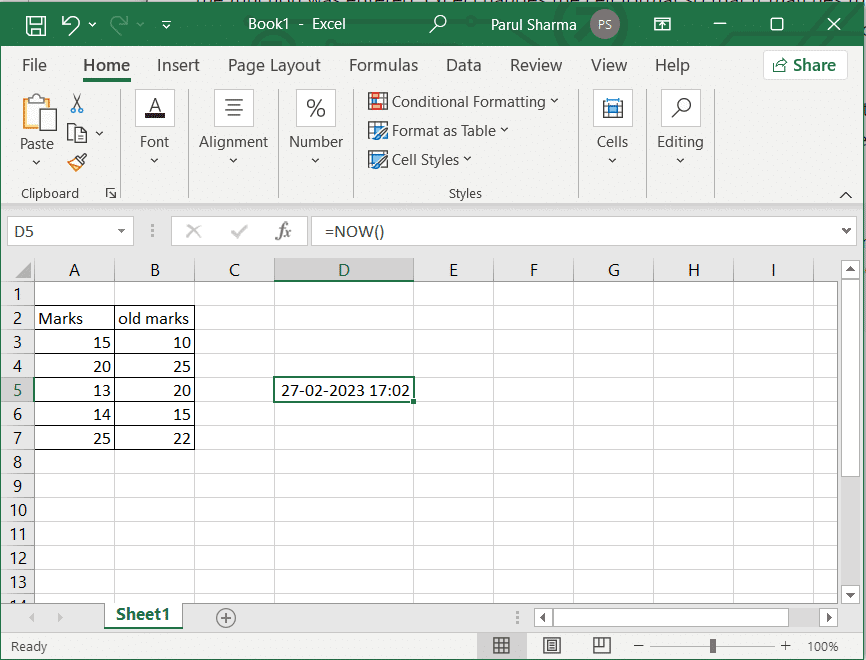#### 6. TODAY

You can use this function to find out the current system date.

=TODAY()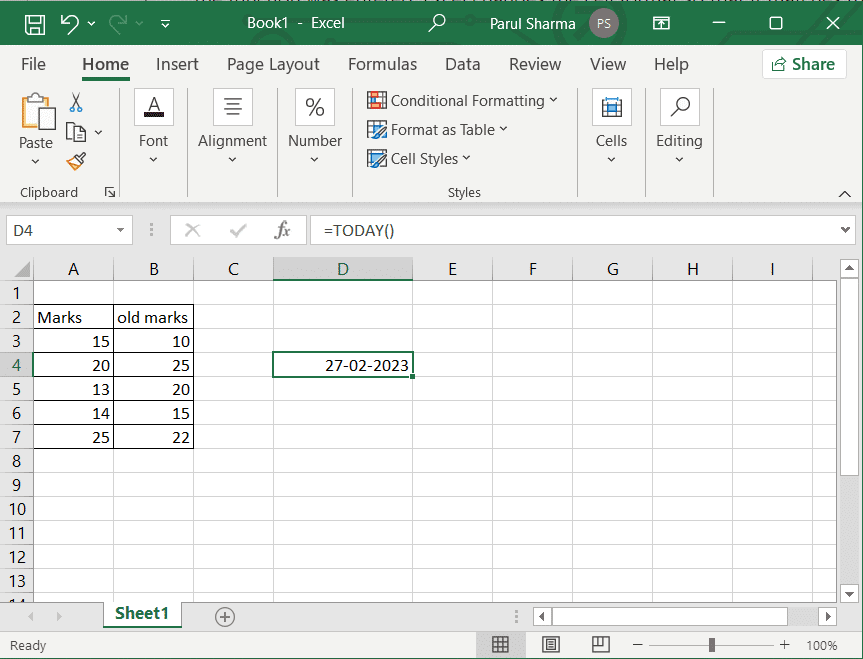Also Read: How to Calculate Z Score in Excel

#### 7. MODULUS

When a number is divided by a divisor, this function works on returning its reminder.

= MOD(number, divisor)

• Number– It is the number for which you wish to find the remainder.
• Divisor– This is the number by which you want to divide the Number.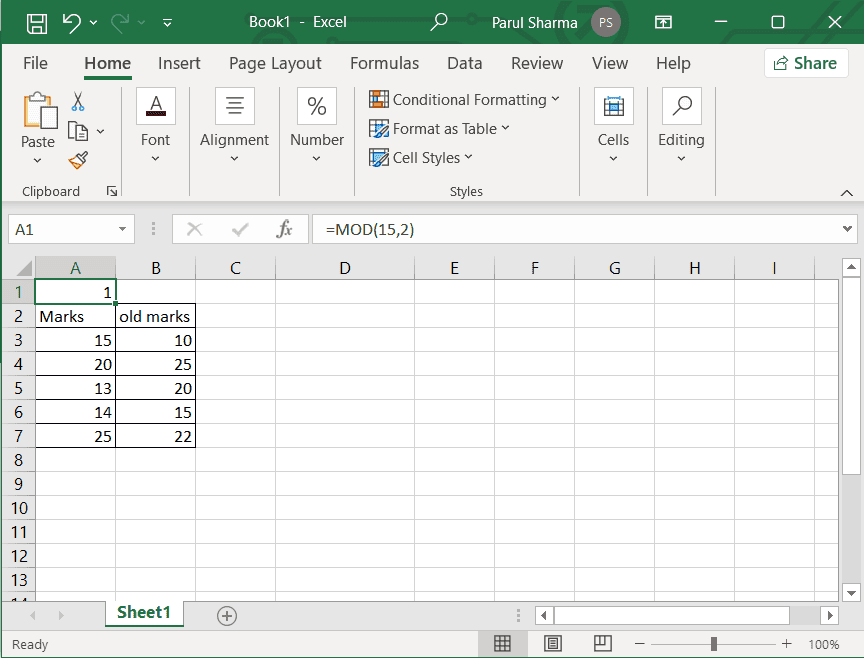#### 8. POWER

This one of the basic 10 functions of MS Excel returns the result of a certain number when raised to a power.

POWER(number, power)

Number– It is the base number which can be any real number.

Power– This is the exponent to which the base number will be raised.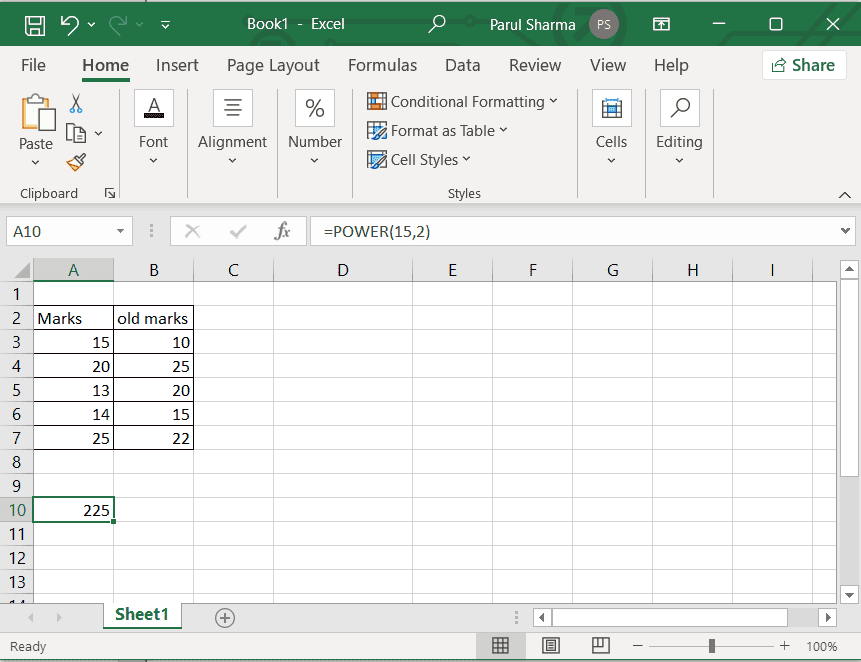#### 9. COUNTIF

This is used to count the total number of cells within a range that meet the required condition.

COUNTIF(Where do you want to look, what are you looking for)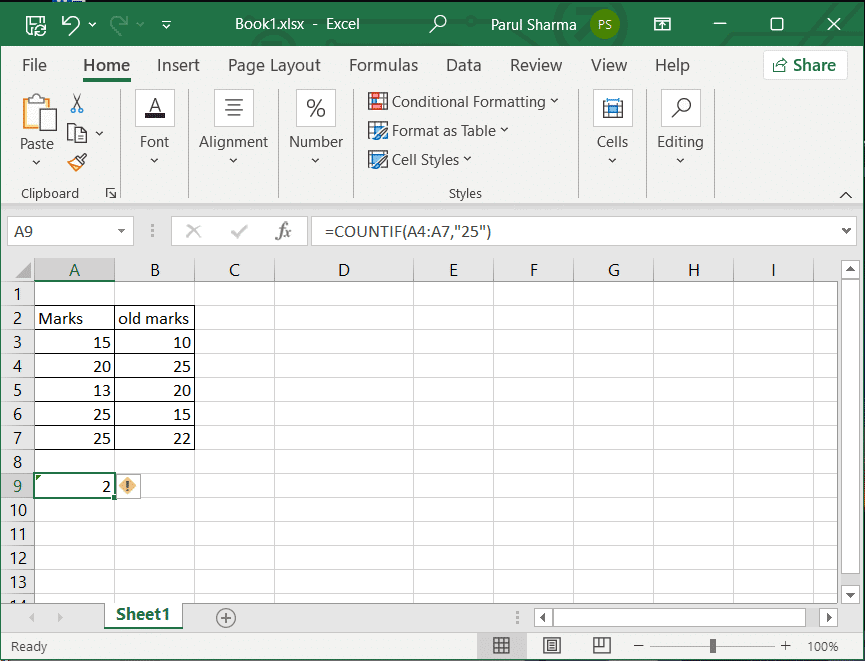#### 10. SUMIF

The SUMIF function adds the values in a range that meet the specified criteria.

SUMIF(range, criteria)

Range– This is the range of cells that you want to be evaluated by criteria.

Criteria– This defines the cells which will be added.